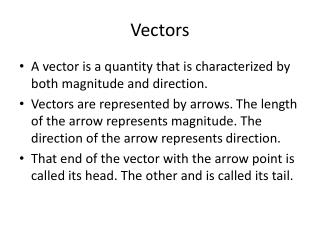DownloadDownload PresentationVectors

# Vectors

Download Presentation## Vectors

- - - - - - - - - - - - - - - - - - - - - - - - - - - E N D - - - - - - - - - - - - - - - - - - - - - - - - - - -
##### Presentation Transcript

1. Vectors • A vector is a quantity that is characterized by both magnitude and direction. • Vectors are represented by arrows. The length of the arrow represents magnitude. The direction of the arrow represents direction. • That end of the vector with the arrow point is called its head. The other and is called its tail.

2. Vectors • Vectors do not have position. A vector may be moved anywhere in a coordinate system, and still be the same vector as long as it's magnitude and direction do not change. • In the next slide all the arrows represent the same vector.

3. Vectors • Symbols representing vector quantities have arrows drawn above them. • In print vectors are frequently represented by boldface characters.

4. Vectors • Vectors are added either graphically or mathematically. • Graphical addition involves placing the tail of one of vector at the head of another, and showing the resultant vector by connecting the tail of the first of vector to the head of the second. • The vector arrows must be drawn very carefully to obtain accurate results.

5. Figure 3-11a (page 61)A + B = B + A

6. Figure 3-11b A + B = B + A

7. Vectors • Multiple vectors may be added into this manner.

8. Figure 3-9 (p. 60)Adding Several Vectors

9. Vectors • In order to represent the negative of a vector, we reverse the head and tail positions.

10. Figure 3-14a Vector Subtraction

11. Vectors • To add and subtract vectors mathematically, we must define a coordinate system, and apply trigonometric methods.

12. Figure 3-3 A Two-Dimensional Coordinate System

13. Vectors • Any vector can be resolved into components. When the components are added together, the result is the original vector.

14. Vectors • It is convenient to resolve vectors into components that lie along the axes of the coordinate system.

15. Vectors

16. Vectors • Standard angle. A standard angle has its vertex at the origin and is measured from the positive x axis. If measured counterclockwise from the positive x axis, the angle is considered positive. If measured clockwise from the positive x axis the angle is considered negative.

18. Vectors • To resolve a vector into perpendicular components, we multiply its magnitude by the appropriate trigonometric function of the angle between the vector and the horizontal axis.

19. Figure 3-7a Vector Angle

20. Vectors • If the angle is between the vector and the vertical axis, different trigonometric functions must be used.

21. Figure 3-7b Vector Angle

22. Figure 3-5 A Vector Whose x and y Components Are Positive

23. Figure 3-6ab Examples of Vectors with Components of Different Signs

24. Figure 3-6cd Examples of Vectors with Components of Different Signs

25. Vectors • Unit vectors. Unit vectors are vectors with a magnitude of 1, that indicate the direction of a vector. The unit vectors that indicate the direction along the axes of a three dimensional cartesian coordinate system, are

26. Vectors • Unit vectors. Another frequently used system of notation for unit vectors is indicates direction along the x axis indicates direction along the y axis indicates direction along the z axis

27. Figure 3-7a Vector Angle

28. Vectors • Unit vectors. To express the above vector using unit vectors we write it as follows. The above expression is the vector in Cartesian coordinates.

29. Vectors Unit vectors • Adding vectors -to add two or more vectors, we resolve them using unit vectors as described above, add the terms multiplied by together, and add the terms multiplied by together. The resultant vector is expressed in Cartesian coordinates.

30. Vectors Unit vectors • To convert the resultant vector to polar coordinates (magnitude and angle), we use the Pythagorean theorem to obtain the magnitude, and the to obtain the angle θ.